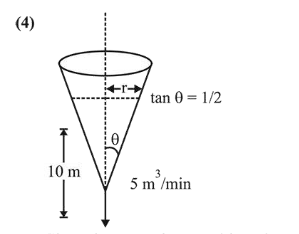# A water tank has the shape of an inverted right circular cone,

Question:

A water tank has the shape of an inverted right circular cone, whose semi-vertical angle is $\tan ^{-1}\left(\frac{1}{2}\right)$. Water is

poured into it at a constant rate of 5 cubic meter per minute. Then the rate (in $\mathrm{m} / \mathrm{min}$.), at which the level of water is rising at the instant when the depth of water in the tank is $10 \mathrm{~m}$; is:

1. (1) $1 / 15 \pi$

2. (2) $1 / 10 \pi$

3. (3) $2 / \pi$

4. (4) $1 / 5 \pi$

Correct Option:

Solution:'

Given that water is poured into the tank at a constant rate of $5 \mathrm{~m}^{3} /$ minute.'

$\therefore \frac{d v}{d t}=5 \mathrm{~m}^{3} / \mathrm{min}$'

Volume of the tank is,

$V=\frac{1}{3} \pi r^{2} h$    ..............(1)

where $r$ is radius and $h$ is height at any time.

By the diagram,

$\tan \theta=\frac{r}{h}=\frac{1}{2}$

$\Rightarrow h=2 r \Rightarrow \frac{d h}{d t}=\frac{2 d r}{d t}$ .........(2)

Differentiate eq. (i) w.r.t. ' $t$ ', we get

$\frac{d V}{d t}=\frac{1}{3}\left(\pi 2 r \frac{d r}{d t} h+\pi r^{2} \frac{d h}{d t}\right)$

Putting $h=10, r=5$ and $\frac{d V}{d t}=5$ in the above equation.

$5=\frac{75 \pi}{3} \frac{d h}{d t} \Rightarrow \frac{d h}{d t}=\frac{1}{5 \pi} \mathrm{m} / \mathrm{min}$